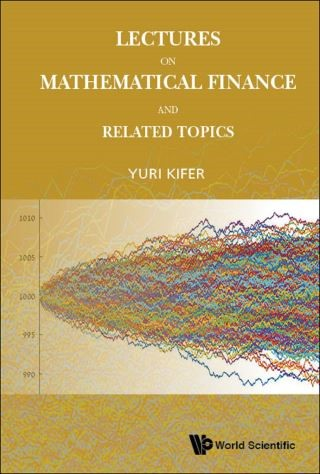## Lectures on Mathematical Finance and Related Topics• Description
• Author
• Info
• Reviews

### Description

Rigorous mathematical finance relies strongly on two additional fields: optimal stopping and stochastic analysis. This book is the first one which presents not only main results in the mathematical finance but also these 'related topics' with all proofs and in a self-contained form. The book treats both discrete and continuous time mathematical finance. Some topics, such as Israeli (game) contingent claims, and several proofs have not appeared before in a self-contained book form. The book contains exercises with solutions at the end of it and it can be used for a yearlong advanced graduate course for mathematical students.

Contents:
• Preface
• Discrete Time:
• Martingales and Optimal Stopping
• Derivatives in General and Binomial Markets
• Fundamental Theorems of Asset Pricing
• Superhedging
• Hedging with Risk
• Continuous Time:
• Martingales in Continuous Time and Optimal Stopping
• Introduction to Stochastic Analysis
• Derivatives in the Black–Scholes Market
• Further Topics:
• Discrete Time Case
• Continuous Time Case
• Solutions of Exercises
• Bibliography
• Index

• Readership: It can be used for a yearlong advanced graduate course for mathematical students.Mathematical Finance;Pricing of Derivatives;Optimal Stopping;Stochastic Analysis0Key Features:
• This is the first self-contained book on the high level which includes "related topics " such as optimal stopping and stochastic analysis, as well as some topics which appeared before only in the periodic literature, such as Israeli (game) contingent claims
• This is the first completely self-contained book (modulo standard probability and measure theory courses) on mathematical finance which includes also a detailed treatment of optimal stopping and stochastic analysis necessary for a self-contained presentation of mathematical finance
• Some topics and proofs from this book appeared before only in the periodic literature